## QCD

### Why Colour?

There are several strong evidences why quarks should carry - besides the electric charge - an additional internal degree of freedom: the colour charge.
1. Quark Wave Function of Baryons
2. Electron Positron Annihilation into Hadrons
Electron-positron annihilation into hadrons provides strong arguments that
* the primary process is electron positron-annihilation into quark-antiquark pairs where
* quarks are spin-1/2 fermions
* that carry electric charges 2/3e or 1/3e, respectively,
* and three different colour charges.

1) Measuring the differential cross section for electron-positron annihilation into hadrons (due to the production of quark-antiquark pairs) was used to show that quarks carry spin 1/2:
Quarks do not exist as free particles but do "hadronize" when produced in a high-energy interaction. Hence, one measures the differential cross section for electron-positron annihilation into hadrons instead.

If the energies of the quark and antiquark in the final state is large the hadrons formed will carry momenta pointing preferentially in the same direction as the primary quark or antiquark. Typical transverse momenta of the hadrons in the hadronization process are O(300 MeV), about the inverse of the typical size of hadrons (O(1 fm)).

To "find" the emission direction of the primary quarks from the emerging hadrons, hadron jets are searched for by defining a "thrust" axis.

This is depicted in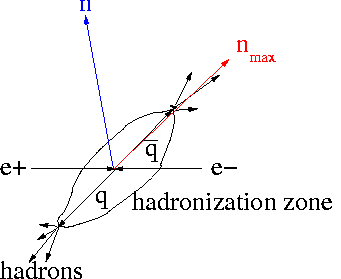One considers an arbitrary unit vector n from which one defines the quantity t(n):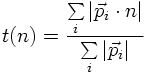The thrust T is the maximum of t(n) with respect to n: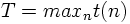The unit vector nmax which maximizes t(n), that is the longitudinal momenta, is called the thrust axis.

The angle of the thrust axis nmax with respect to the beam axis for two back-to-back hadron jets shows the same QED-dependence as the annihilation into a muon-antimuon pair: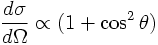This strongly supports the idea that primary spin-1/2 quark-antiquark pairs are produced in electron-positron annihilations subsequently hadronizing into hadrons which are then measured in the detector.

2) While the integrated cross section for hadron final states in electron-positron annihilation shows in general the QED 1/s-behaviour typical for pointlike particles there are some prominent peaks at located CMS energies.

At these energies hadron resonances are produced which carry the same quantum numbers as the photon: JPC=1--. That is, these resonances are directly produced by a virtual s-channel photon and decay subsequently into hadrons. These hadron resonances contain quark-antiquark pairs. The prominent ones are: ρ0, ω, φ, J/Ψ, Υ. The dependence of the annihilation cross section as a function of the CMS energy can be found in here.

The additional peak close to 90 GeV is caused by the production of a real Z0 boson.

3) An important observable as a function of the CMS energy is the ratio of cross sections R: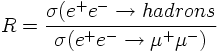QUESTION: Could one also use an electron-positron in the final state for the nomrlization reaction in the denominator?

If the quarks cary a charge of Qqe (Q=2/3 or 1/3) and if the quarks carry also the internal degree of freedom 'Colour' with tree charge values 'blue', 'red' and 'green' the ratio R is predicted to be: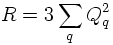if masses can be neglected.

The measured ratio R (also found in here) strongly supports that quarks carry charges Q=2/3 (u, c) and Q=1/3 (d, s, b) and in addition carry 'Colour'.

In summary: electron-positron annihilation into hadrons strongly supports that
* hadrons contain pointlike charged partons (constancy of R)
* these partons carry spin-1/2 (angular distribution of hadron jets)
* these partons carry fractional charges and the colour quantum number (values for R)

These facts are consistent with the quark hypothesis.

3. tau decays
4. pi0 decay